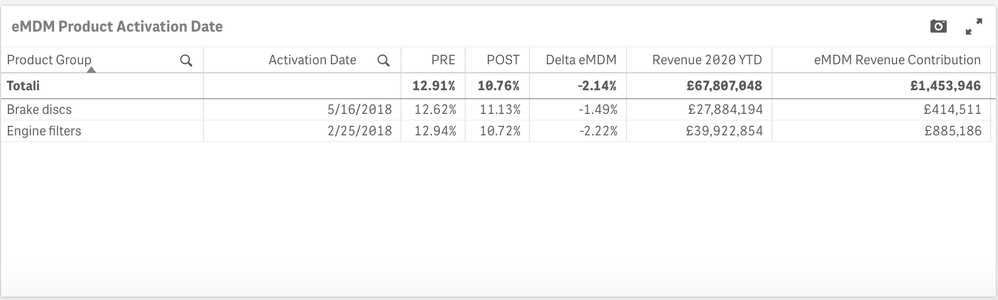# New to Qlik Sense

If you’re new to Qlik Sense, start with this Discussion Board and get up-to-speed quickly.

Announcements
Skip the ticket, Chat with Qlik Support instead for instant assistance.
cancel
Showing results for
Did you mean:Contributor

## Individual row sum

I want to calculate a KPI which is an aggregation of row-wise data taken individually.

For example –eMDM Revenue contribution is the column I am looking for.

The total should be 414,511 + 885,186 = 1,299,697

But the total is being calculated for Delta eMDM * Revenue 2020 YTD, i.e.,

2.14% of 67,807,048 = 1.4M

How to achieve this?

I am using this in KPI. The KPI works fine when I select an individual product group but is wrong without selection as Qlik is calculating on totals.

Formulas used –

Delta eMDM = Post - Pre

((sum(aggr(Sum({\$<Year={2020}>} Return_eMDM_POST),Product_Name))

/

sum(aggr(Sum({\$<Year={2020}>} Invoice_POST),Product_Name)))

-

(sum(aggr(sum(Return_eMDM_PRE),Product_Name)) / sum(aggr(sum(Invoice_PRE),Product_Name))))

Revenue 2020 YTD =

Sum(aggr(sum({\$<Year={2020}>} Revenue_PRE),Product_Name)) + Sum(aggr(sum({\$<Year={2020}>} Revenue_POST),Product_Name))

eMDM revenue Contribution

( (sum(aggr(Sum({\$<Year={2020}>} Return_eMDM_POST),Product_Name))

/

sum(aggr(Sum({\$<Year={2020}>} Invoice_POST),Product_Name)))

-

(sum(aggr(sum(Return_eMDM_PRE),Product_Name)) / sum(aggr(sum(Invoice_PRE),Product_Name))))

*-

(Sum(aggr(sum({\$<Year={2020}>} Revenue_PRE),Product_Name)) + Sum(aggr(sum({\$<Year={2020}>} Revenue_POST),Product_Name)))

It is just a multiplication of the above 2 formulas.

Labels (1)
• ### Qlik Sense

0 RepliesTags
Community Browser